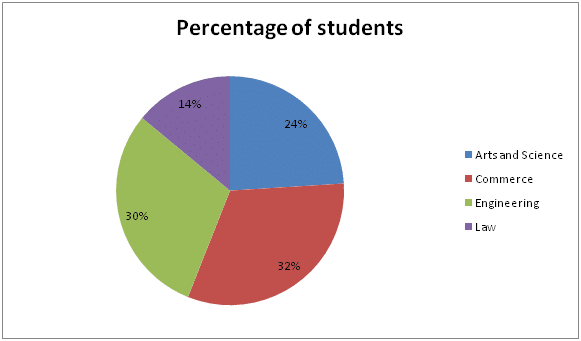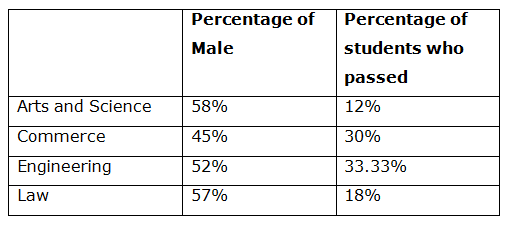# SBI Clerk Pre Quantitative Aptitude (Day-37)

Dear Aspirants, Our IBPS Guide team is providing new series of Quantitative Aptitude Questions for SBI Clerk Prelims 2020 so the aspirants can practice it on a daily basis. These questions are framed by our skilled experts after understanding your needs thoroughly. Aspirants can practice these new series questions daily to familiarize with the exact exam pattern and make your preparation effective.

Start Quiz

Ensure Your Ability Before the Exam – Take SBI Clerk 2020 Prelims Free Mock Test

Simplification

Directions (1 – 5): What value should come in place of (?) in the following questions?

1) (7 (1/7) – 5 (1/5)) × 5 (5/6) ÷ 5 (5/16) + 26/30 = √(? – 62)

a) 68

b) 75

c) 71

d) 82

e) 78

2) 5.6% of 350 + 12.5% of 480 = ?2 – 17% of 120

a) 12

b) 10

c) 6

d) 8

e) 11

3) (14)7 × (16)-4  ÷ ((343)2 × (128)-2) = 2 × 7 × √x

a) 16

b) 64

c) 256

d) 1024

e) 4

4) (2 ½ % of 160) × (21/4 % of 110) × 9 = 16 × 15 – 1/10 – (2)?

a) 4

b) 3

c) 5

d) 6

e) 8

5) (2 (1/4) * 18/63 + 63/90 * 2 (2/49)) / (3/7 + 4/9 + 1/21) =?/64

a) 72

b) 128

c) 216

d) 64

e) 144

Data Interpretation

Directions (6 – 10): Study the following information carefully and answer the given questions?

The following pie chart represents the number of students from different streams who attended an exam.

Total number of students = 800000The following table shows the percentage of male in different streams and the percentage of students who passed the examination.6) What is the ratio of number of females from Arts and Science to the number of females from Commerce?

a) 32:55

b) 30:53

c) 63:110

d) 67:110

e) 29:110

7) What is the total number of students who passed the examination?

a) 190000

b) 199992

c) 196754

d) 205606

e) 202000

8) From engineering students, 25% of female passed the examination. Then find the approximate percentage of male students who passed the examination?

a) 41%

b) 50%

c) 33%

d) 27%

e) 58%

9) Find the difference between the number of males from Engineering and law together and the number of females from Engineering and law together?

a) 35820

b) 30270

c) 26870

d) 25280

e) 32850

10) Find the percentage of male students who write the examination?

a) 49.6%

b) 52.9%

c) 53.2%

d) 47.1%

e) 51.9%

Directions (1 – 5):

50/7 – 26/5 = (250 – 182)/35 = 68/35

68/35 * 35/6 * (16/85) = 32/15

32/15 + 26/30 = 90/30 = 3

Given that,

3 = √(x – 62)

x = 9 + 62 = 71

5.6% of 350 + 12.5% of 480 = x2 – 17% of 120

5.6 * 3.5 + 12.5 * 4.8 = x2 – 17 * 1.2

19.6 + 60 = x2 – 20.4

x2 = 100

x = 10

(14)7 * (16)-4 / ((343)2 * (128)-2) = 2 * 7 * √x

27 * 77 * (24)-4 / (76 * (27)-2) = 2*7*√x

2(7-16+14) * 7(7-6) = 2*7*√x

25 * 7 = 2*7*√x

24 = √x

x = 256

(2 1/2% of 160) × (21/4% of 110) × 9 = 16 × 15 – 1/10 – (2)?

5/200 * 160 * 21/400 * 110 * 9 = 240 – 1/10 – (2)x

207.9 = 240 – 0.1 – (2)x

(2)x = 240 – 0.1 – 207.9

(2)x = 32

(2)x = 25

x = 5

(2 (1/4) * 18/63 + 63/90 * 2 (2/49)) / (3/7 + 4/9 + 1/21) = x/64

(9/4 * 18/63 + 63/90 * 100/49) / (3/7 + 4/9 + 1/21) = x/64

(9/14 + 10/7) / (27 + 28 + 3/63) = x/64

(29/14) / (58/63) = x/64

29/14 * 63/58 * 64 = x

144 = x

Directions (6 – 10):

Number of students from Arts and Science = (24/100) * 800000 = 192000

Number of females from Arts and Science = (42/100) * 192000 = 80640

Number of students from Commerce = (32/100) * 800000 = 256000

Number of females from Commerce = (55/100) * 256000 = 140800

Ratio = 80640: 140800 = 63: 110

Number of students passed from

Arts and Science = (12/100) * 192000 = 23040

Commerce = (30/100) * 256000 = 76800

Engineering = (33.33/100) * (30/100) * 800000 = 79992

Law = (18/100) * (14/100) * 800000 = 20160

Total number of students who passed = 199992

Engineering

Number of students = (30/100) * 800000 = 240000

Number of male = (52/100) * 240000 = 124800

Number of female = (48/100)*240000 = 115200

25% of female passed = (25/100)*115200 = 28800

Total number of students passed = (33.33/100) * 240000 = 79992

Number of male passed = 79992 – 28800 = 51192

Percentage of male students passed = (51192 / 124800) * 100 = 41%

Engineering

Number of males = 124800

Number of females = 115200

Law

Number of students = (14/100)*800000 = 112000

Number of male = (57/100)*112000 = 63840

Number of female = 112000 – 63840 = 48160

Number of males from Engineering and law = 124800 + 63840 = 188640

Number of females from Engineering and law = 115200 + 48160 = 163360

Difference = 188640 – 163360 = 25280

Number of male

Arts college = (58/100) * 192000 = 111360

Commerce = (45/100) * 256000 = 115200

Engineering = (52/100) * 240000 = 124800

Law = (57/100) * 112000 = 63840

Total males = 111360 + 115200 + 124800 + 63840 = 415200

Percentage = (415200 / 800000) * 100 = 51.9 %

 Check Here to View SBI Clerk Prelims 2020 Quantitative Aptitude Questions Day 36 Day 35 Day 34 Click Here for SBI Clerk 2020 – Detailed Exam Notification